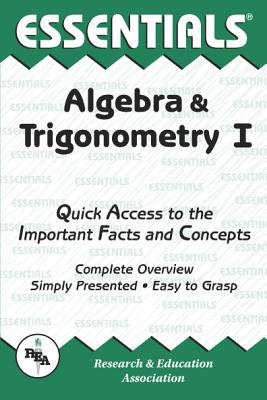### Algebra & Trigonometry I Essentials (Paperback)

Research & Education Association, 9780878915699, 96pp.

Publication Date: October 23, 1987

List Price: 6.95*
* Individual store prices may vary.

#### Description

REA's Essentials provide quick and easy access to critical information in a variety of different fields, ranging from the most basic to the most advanced. As its name implies, these concise, comprehensive study guides summarize the essentials of the field covered. Essentials are helpful when preparing for exams, doing homework and will remain a lasting reference source for students, teachers, and professionals. Algebra & Trigonometry I includes sets and set operations, number systems and fundamental algebraic laws and operations, exponents and radicals, polynomials and rational expressions, equations, linear equations and systems of linear equations, inequalities, relations and functions, quadratic equations, equations of higher order, as well as ratio, proportion, and variation.